Email   Home Page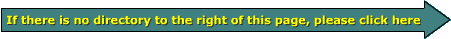Resistors Resistors are passive electronic devices that either limit current or allow for a drop in voltage. This page will cover some of the most common types of resistors and will show you how they work in a circuit. Schematic Symbols: These are 2 generic schematic symbols for a resistor. The symbol shaped like a resistor is more common on schematics used in Europe and Asia. The bottom symbol is more common in the US.Resistors as Current Limiting Devices: Generally, a power supply isn't specifically designed to drive a single device. In most cases, an electronic device (such as an amplifier or a head unit) has a power supply and all of the components operate from that supply. The supplies can deliver enough current for all of the circuits. If any one part of the circuit were connected to the supply without some current limiting device, that part of the circuit would likely be destroyed. For example, let's say we have a supply that's capable of delivering 30 amps of current. Let's also say that we need to power an LED (light emitting diode). If the LED were connected directly to the supply, it would be destroyed instantly. Adding a current limiting device such as a resistor limits the current flow through the LED and allows the LED to operate properly. Resistors as Voltage Dividers: Sometimes, only a fraction of the the full power supply voltage is needed at a given point in a circuit. If two resistors are connected in series between the power supply output terminals, the voltage on the point where the resistor connect to each other will be a fraction of the total voltage. By varying the values of the resistors, you can vary the voltage between the resistors. This will be discussed in detail later on this page. The potentiometers page will discuss voltage division in great detail. Resistor Specifications: Resistors have two main parameters. The first is its resistance in ohms. The second is its power rating in watts. There are other specs such as maximum working voltage but its power rating and resistance value are the most important in car audio systems. Note: In the diagrams below we are considering the battery to be ideal. This means that it has no internal resistance and always at a constant 12 volts. If an ideal piece of wire (no resistance) is connected to the ideal battery (no internal resistance) as shown by the orange line, there would be an infinite amount of current flow through the wire. In the real world, the battery (like your car battery) would force enough current through the wire and create enough heat in the wire to, at the very least, melt/burn the insulation off of the wire and more than likely incinerate anything that comes in contact with it. If the wire were so large that the battery could not cause it to overheat, the battery would likely overheat and fail. This type of short circuit can easily cause 500+ amps of current to flow. For large batteries like the Odyssey PC2250, the current flow from a short circuit could be as much as 5000 amps. ----- Critically Important ----- Adobe has deemed that the Flash content on web pages is too risky to be used by the general internet user. For virtually all modern browsers, support for Flash was eliminated on 1-1-2021. This means that those browsers will not display any of the interactive Flash demos/calculators/graphics on this (or any other) site. The simplest (not the best) fix, for now, is to download the Ruffle extension for your browser. It will render the Flash files where they were previously blocked. In some browsers, you will have to click on the big 'play' button to make the Flash applets/graphics visible. An alternative to Ruffle for viewing Flash content is to use an alternative browser like the older, portable version of Chrome (chromium), an older version of Safari for Windows or one of several other browsers. More information on Flash capable browsers can be found HERE. It's not quite as simple as Ruffle but anyone even moderately familiar with the Windows Control Panel and installation of software can use Flash as it was intended. If a resistor is connected to a battery like shown in the above diagram, there would be less current flow than in the wire alone. How much less current depends on the value of the resistor. If the value of the resistor is 1 ohm and the battery voltage is 12 volts, according to Ohm's law the current through the resistor is 12 amps. I=E/R Which means current flow is equal to the applied voltage divided by the resistance in the circuit. I=12/1 12 volts applied to a 1 ohm resistor I=12 amps Current flow through the resistor is 12 amps. If the resistor's value is 10 ohms, with the same 12 volts applied to it, then the current flow will be less (because the resistor presents more resistance to the flow of current). The current flow will be 1.2 amps. I=E/R I=12/10 12 volts applied to a 10 ohm resistor I=1.2 amps Current flow through the resistor is 1.2 amps. If you were trying to determine the power rating needed for either resistor, you could use one of three formulae. P=I*E or P=E^2/R or P=I^2*R I will show you 2 examples here. Using the formula P=I*E, you can see that the power being dissipated by the resistor is a product of the current and the applied voltage. For the 1 ohm resistor, the power dissipation is: P=I*E P=12*12 P=144 watts If you didn't already know the current flow through the 1 ohm resistor, you could use the formula P=E^2/R. P=E^2/R P=(12*12)/1 P=144 watts This may not seem like much power but if the air flow around the resistor is restricted, it will become very hot. The 1 ohm resistor would have to be rated at 144 watts or higher to prevent its failure (from the heat generated in the resistive element). Using the formula P=I*E, for the 10 ohm resistor, the power dissipation is: P=I*E P=1.2*12 P=14.4 watts Using the formula P=E^2/R, for the 10 ohm resistor, the power dissipation is: P=E^2/R P=(12*12)/10 P=14.4 watts The 10 ohm resistor would have to be rated at 14.4 watts or higher to prevent it from dying a horrible painful death. Resistor Color Codes: The most common resistors have a tolerance of plus or minus 5%. These resistors have 4 color bands. The first 2 color bands give the first 2 digits of the resistor's value. The 3rd band give the number of 0's added to the first 2 digits. If the third band is gold, you multiply the first 2 digits by .1. If it is silver, multiply by .01. The fourth band gives the tolerance. Gold is a 5% tolerance. Silver is 10%. The tolerance band is generally set off from the other bands. The other bands are generally set close together. The list below shows the values for the resistor color codes of non-precision resistors. Band 5 is rare in car audio equipment but I'll include the info anyway. The reliability of a resistor indicates the failure rate of a resistor when run at its rated power dissipation for 1000 hours.

 Resistor Color Codes Band 1 Band 2 Band 3 Band 4 Band 5 Color 1st Digit 2nd Digit Multiplier Tolerance Reliability Black 0 1 Brown 1 1 10 1% Red 2 2 100 0.1% Orange 3 3 1,000 0.01% Yellow 4 4 10,000 0.001% Green 5 5 100,000 Blue 6 6 1,000,000 Violet 7 7 10,000,000 Gray 8 8 100,000,000 White 9 9 1,000,000,000 Gold x 0.1 5% Silver x 0.01 10%

The image below is a 3300 ohm 5% resistor.1st band Black Brown Red Orange Yellow Green Blue Violet Gray White
 2nd band Black Brown Red Orange Yellow Green Blue Violet Gray White
 Multiplier band Black Brown Red Orange Yellow Green Blue Violet Gray White
 Multiplier band continued Gold Silver Tolerance band Gold Silver Ohms Tolerance ± Ohms

 The following chart shows what each of the bands means on a close tolerance resistor. They have an additional band which allows an additional significant digit for the value of the resistor.

 Precision Resistor Color Codes Band 1 Band 2 Band 3 Band 4 Band 5 Band 6 Color 1st Digit 2nd Digit 3rd Digit Multiplier Tolerance Reliability Black 0 0 1 Brown 1 1 1 10 1% 1% Red 2 2 2 100 2% 0.1% Orange 3 3 3 1,000 3% 0.01% Yellow 4 4 4 10,000 0.001% Green 5 5 5 100,000 Blue 6 6 6 1,000,000 Violet 7 7 7 10,000,000 Gray 8 8 8 100,000,000 White 9 9 9 1,000,000,000 Gold x 0.1 5% Silver x 0.01 10%

5 Band Resistor Calculator

The image below is a 3300 ohm 1% resistor.1st band Black Brown Red Orange Yellow Green Blue Violet Gray White
 2nd band Black Brown Red Orange Yellow Green Blue Violet Gray White
 Third band Black Brown Red Orange Yellow Green Blue Violet Gray White
 Multiplier band Black Brown Red Orange Yellow Green Blue Violet Gray White
 Multiplier band continued Gold Silver 5th band Brown Red Orange Gold Silver Ohms Tolerance ± Ohms

 Resistor Wattage Ratings: The photo below shows various styles and wattage resistors. From left to right, the wattage ratings are: 5 watt, 3 watt, 2 watt, 1 watt, 1/2 watt, 1/4 watt and a 1/6 watt.Common resistor wattage ratings are: 1/8w, 1/4w, 1/2w, 1w, 2w, 3w, 5w, 10w. There are others but these are the ones that you will likely need to use.
 Use this program to calculate the power dissipation in a resistor This calculator will show you how the voltage applied to a resistor and the resistor's value determine the power dissipated in a resistor. You may manually enter the the voltage and resistance.

 Input ohms volts Output Power Dissipation = Watts Current = Amps

Note: In the diagrams below we are considering the battery to be ideal, no internal resistance and always at a constant 12 volts. Current flow will be 'conventional' flow (positive to negative).

Resistors as Voltage Dividers: You already know that a resistor can be used to limit the current flow in a circuit. When multiple resistors are used in series, they will divide the voltage from the power supply (a battery in this example). In this first diagram you can see that the voltage across the resistor is the same as the voltage across the battery.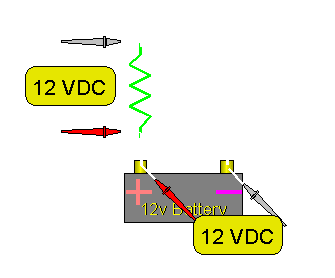Equivalent Circuits: The following 3 circuits are identical. Don't let the different configurations confuse you.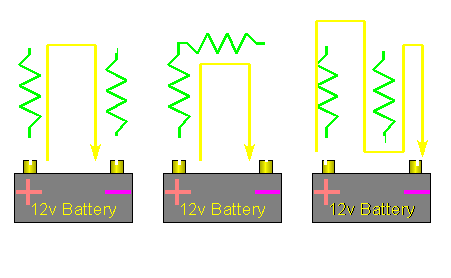2 Resistors in Series: The resistors in the following diagram are in series. Since they are the same value (1000 ohms), the voltage drop across each resistor is the same. Each resistor drops half the supply voltage (6 volts).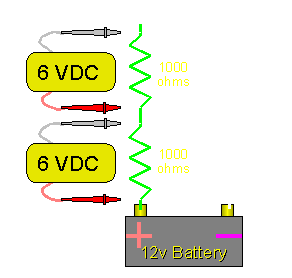3 Resistors in Series: If there were 3 equal value resistors, the voltage would be divided equally between them. They would each have a voltage drop of 4 volts (3*4=12). As you can see, the voltage drop across all of the resistors will add up to the power supply voltage.

Different Value Resistors: If the resistor values are different, you can still calculate the voltage difference across the resistors. There are a few different ways to calculate the voltage. I'll show you the most versatile way. This is the circuit: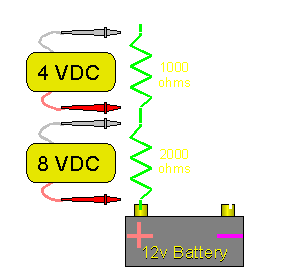We know:

• The total voltage
• The individual resistor values

We can calculate the current flow and then the voltage drop across the individual resistors. From the Ohm's Law page, we will use the formula:
I = V/R
I = 12/3000 ohms
I = 0.004 amps or 4 milliamps

The current flow through the resistors is 4 milliamps. Since the resistors are in series, we know that the current flow through each resistor is the same.

Then, to find the voltage drop across the 1000 ohm resistor, we can use the formula:
V = I*R
V = .004*1000
V = 4 volts across the 1000 ohm resistor

And to find the voltage drop across the 2000 ohm resistor, we can use the formula:
V = I*R
V = .004*2000
V = 8 volts across the 2000 ohm resistor

The previous method (using current flow to calculate voltage drop) will work for any number of series connected resistors. There is another method to find the voltage drop across a resistor when there are only 2 resistors. The formula is:
V = (R1/(R1+R2))*battery voltage
For the following diagram, these are the calculations:
V = (4700/(4700+2200))*12
V = 8.17 volts across the 4700 ohm resistor.Using Resistors to Increase an Amplifier's Power Output

As we found earlier on this page, a resistor can be used to dissipate power. Some people believe that they will have an increase in system SPL if they reduce the amplifier load's impedance with resistors. The fact is, the SPL will likely be reduced. Just because the amplifier is producing more power, it does NOT mean the SPL will increase. The reason? The extra power is dissipated in the form of heat and produces no audio. The reason that the SPL will likely drop is because the amplifier's internal power supply will lose some rail voltage with the lower impedance load (the loss may not be significant on amps with highly regulated power supplies). When the rail voltage drops, the output power to the speaker drops. Even if you have an amplifier with a regulated power supply and the power to the speakers doesn't fall, the amplifier will draw more current and run hotter.

• In the following diagram, you can see a few different things:
• You can see that the amplifier is producing almost twice the power with the resistor in parallel with the load.
• The rail voltage is reduced with the heavier load.
• The power to the speaker is reduced because less rail voltage is available.
• The current draw has more than doubled. It is more than twice due to inefficiencies within the amplifier.
• The amplifier will run hotter because of more voltage drop across and more current through all of the semiconductors.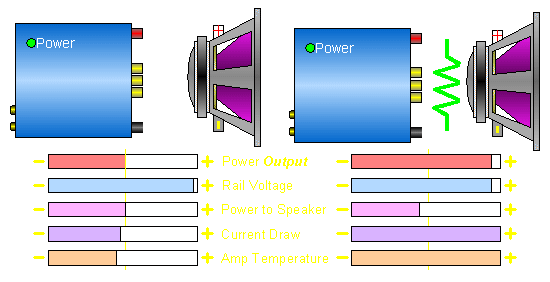Resistor Construction:
There are several different ways to make resistive components. I'll try to cover a few of them here.

Film Resistors:
In the following diagram, you can see a ceramic substrate covered in a resistive film. The substrate is held, on each end, by metallic end caps. The wire leads are welded onto the end caps. Film resistors are (generally) made from etching a resistive element from a film of resistive material. The composition of the resistive film can vary from one type of resistor to another but the following description covers most type of film resistors. The ceramic (or glass) substrate is covered with a resistive material. The resulting component is effectively a relatively low ohm component. To change the component's value, the resistive film is lengthened by cutting a helical grove in it.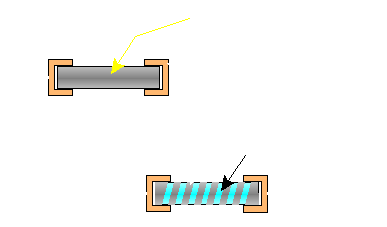The resistance can be varied by varying the way the element is cut. In this diagram, you can see that leaving a wide and relatively short resistive element results in a low ohm resistor. A narrower longer helix results in a higher value resistor.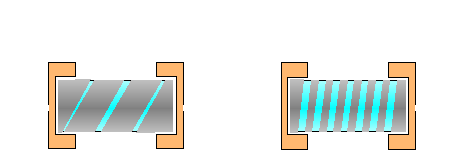Types of Film Resistors

There are several different types of film resistors. The following are a few of their characterisitcs.

Carbon Film Resistors:
Carbon film resistors are some of the least expensive and therefore the most common resistors in use today. They are formed in one of 2 ways. The first is as described above. A carbon film is deposited on the ceramic substrate when the substrate is exposed to hydrocarbon gasses in a vacuum (at high temperatures). The film is then cut to produce the desired resistor value. Another way the carbon film resistor can be formed is by painting a carbon filled polymer onto the former/substrate. The resistor value is determined by the amount of carbon in the polymer, the width and the length of the resistive element. Carbon film resistors are most commonly available in 5% tolerance.

Metal Film Resistors:
Metal film resistors are much like the carbon film resistors but instead of having a carbon material deposited on the former, a metal film such as nichrome is deposited.

Metal Oxide Resistors:
The resistive element in a metal oxide resistor is formed by the process of oxidation of a chemical like tin-chloride on the ceramic substrate. Metal oxide resistors can withstand higher temperatures than metal film or carbon film resistors. They can also better withstand short term surges.

Carbon Composition Resistors

Carbon Composition Resistors:
Carbon composition resistors are formed a little differently that the previously described film resistors. In all of the film resistors, the resistive element has very little thermal mass. If there is a short term surge through the resistor, the small thin element can quickly overheat and fail. In a carbon composition resistor, the resistive element is much thicker and therefore more able to handle short term surges without failing. The following diagram shows how the carbon composition resistor differs from film resistors. The value of the resistor can be controlled by the amount of the carbon in the 'slug'. Due to cost, carbon composition resistors are not used very often in car audio equipment.Wire Wound Resistors:
There are many different styles of wire wound resistors. The 2 most common are the ceramic cased (cement) resistor and the type that look much like a large version of a film resistor. The ceramic type generally have a small element inside of a large casing. The large casing is needed to help dissipate heat and prevent the temperature from getting too high (which would cause the resistor to fail). The other type has the wire wound on top of the former. Many times, the wire is visible as a ridge under the insulating coating.The next resistor is a 7 watt wirewound resistor encased in ceramic. The second image is the resistor with the ceramic removed.The following type of resistor is the type most people know as a high power resistor. These resistors 'can' be used to dissipate a lot of power but they need help to do so. For them to dissipate their rated power, they have to be tightly clamped to a heatsink. Without a suitable heatsink, the resistor can only dissipate a tiny fraction of its rated power. The resistor inside the aluminum housing is much like the resistive element above. These are commonly used as dummy loads for testing amplifiers but I no longer use them. They are much more fragile than the next two resistors. Even with the heatsink, they don't handle power surges well.This is a 25 watt wirewound resistor with solder lugs. Wirewound resistors like this resistor and the next one need no heatsink to dissipate their rated power. These are the type of resistors that I use for dummy loads when testing amplifiers (100+ watt resistors in series/parallel).This next resistor is a 220 watt wirewound resistor. As you can see, it's relatively large but larger resistors are readily available.Flame-Proof Resistors:
Flame-proof resistors are available in several different materials (carbon film and metal film are most common). The main thing that distinguishes a flame-proof resistor from a common resistor is its coating. Most resistors will overheat and burn when too much current flows through them. The coating on a flame-proof resistor will not flame up (although it may turn dark or even black). This type of resistor is very commonly used in the audio section of home amplifiers.

Power Handling:
One of the factors that determines the power rating of a resistor is it's ability to dissipate heat. The maximum temperature that a resistor can withstand, without being damaged, is determined by the materials used in its construction. To prevent the resistor's temperature from getting too high, there has to be enough of a heat sink to soak up and/or dissipate the heat. If the resistor is not mounted onto a heat sink, it's physical size generally determines its power rating. Larger resistors have more surface area and can dissipate heat at a greater rate than smaller resistors. Even some large resistors (like the aluminum resistor above) need an additional heat sink to dissipate its rated power. Without a heat sink, its rated to dissipate only about 10-15 watts. To dissipate 50 watts, a significantly larger heat sink would be required.

You May Be Interested in My Other Sites
• This site was started for pages/information that didn't fit well on my other sites. It includes topics from backing up computer files to small engine repair to 3D graphics software to basic information on diabetes.
• This site introduces you to macro photography. Macro photography is nothing more than the photography of small objects. It can take quite a while to understand the limitations associated with this type of photography. Without help, people will struggle to get good images. Understanding what's possible and what's not possible makes the task much easier. If you need to photograph relatively small objects (6" in height/width down to a few thousandths of an inch), this site will help.
• If you're interested in air rifles, this site will introduce you to the types of rifles available and many of the things you'll need to know to shoot accurately. It also touches on field target competition. There are links to some of the better sites and forums as well as a collection of interactive demos.
• This site helps anyone new to computers and anyone with a basic understanding of computers with a desire to learn more about the internal components of a computer. If you have a computer that you'd like to upgrade but don't know where to start, this is a good site for you.
• This site is for those who want to begin racing karts but don't fully understand how the various parts work. It's mostly interactive demos that show how the various parts of the kart work.

•  You should remember: 1. If a resistor is inserted into a series circuit, the current flow will be reduced. 2. The reduction of current flow is directly proportional to the resistance value of the resistor. 3. When current flows through a resistor, there will be a voltage drop across the resistor. 4. When there is voltage drop across the resistor, there will be power dissipation. 5. Power dissipation will cause a rise in temperature of the resistor.

```

```